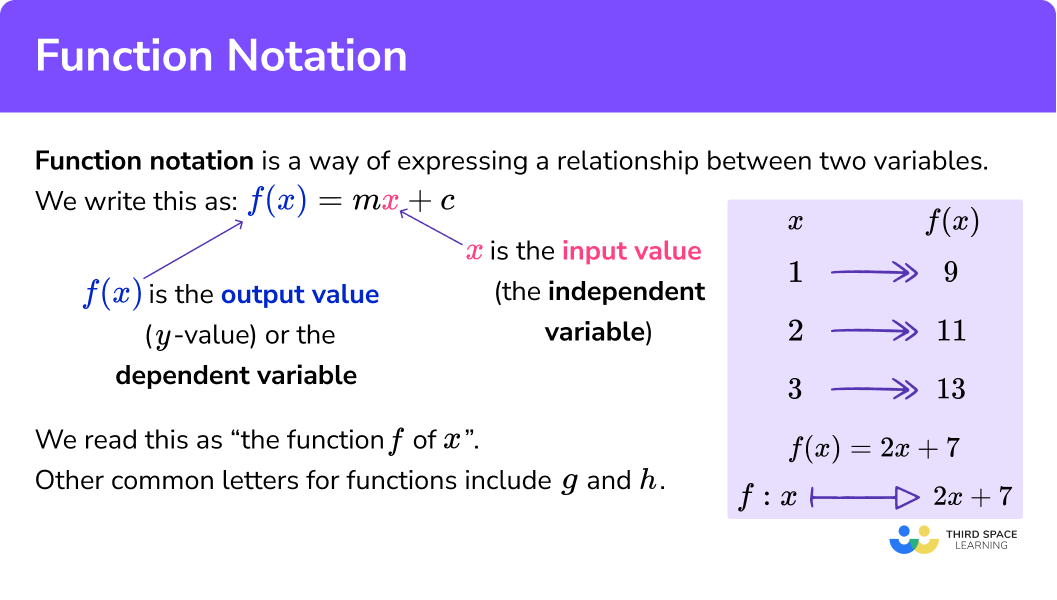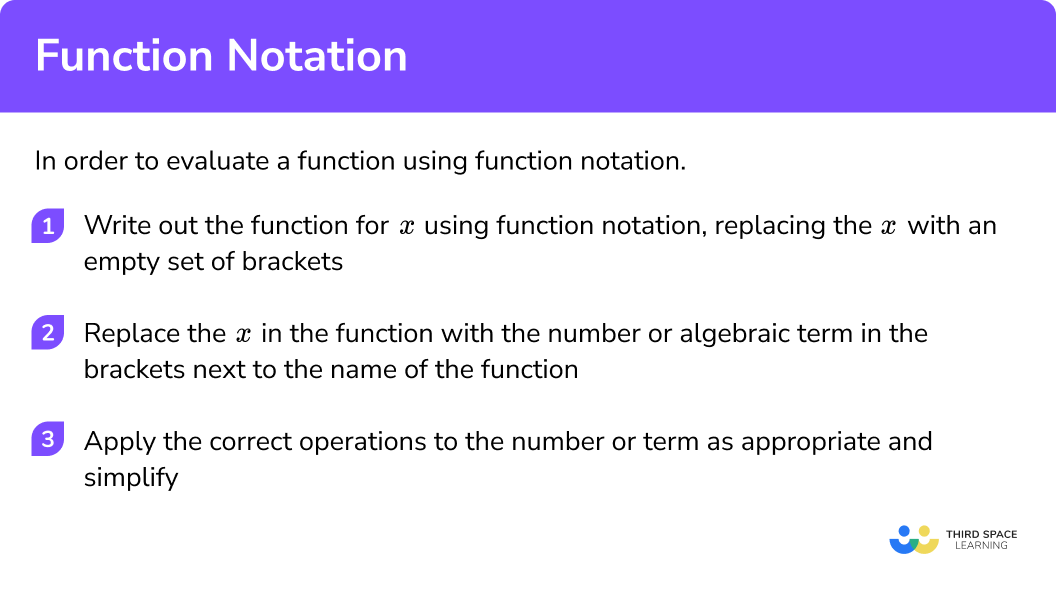GCSE Maths Algebra Functions

Function Notation

# Function Notation

Here we will learn about function notation, including different forms of function notation, how to evaluate functions for given values and how to manipulate algebraic expressions using functions.

There are also function notation worksheets based on Edexcel, AQA and OCR exam questions, along with further guidance on where to go next if you’re still stuck.

## What is function notation?

Function notation is a way of expressing a relationship between two variables.

We are used to writing equations of straight lines in the form y = mx + c .
Using function notation we can write this as f(x) = mx + c , by replacing y with f(x) .
We can read this as “ the function f of x ”.
“f” can be thought of as the “name” of the function. We do not always have to use f for the name of the function; other common names include function g or function h.

The x is the input value known as the independent variable.
f(x) is the output value ( y -value) known as the dependent variable.

To use function notation we just substitute the values of x into the expression and  evaluate it.
The diagram shows the value of a function for different x -values:

There are different types of function notation. As well as examples like g(x) = x^2 + 2 , we may also see it given as g : x → x^2 + 2 . This type of function notation that is more common in A level mathematics.

Function notation is also used in the table function of a scientific calculator. The table function is useful for finding values when graphing linear equations, quadratics, cubics and other polynomials.

### What is function notation?## How to use function notation

In order to evaluate a function using function notation:

1. Write out the function for x using function notation, replacing the x with an empty set of brackets (parentheses).
2. Replace the x in the function with the number or algebraic term in the brackets next to the name of the function.
3. Apply the correct operations to the number or term as appropriate and simplify.

### Explain how to use function notation### Related functions lessons

Function notation is part of the larger topic, functions. You may find it useful to explore the main topic before looking into the detailed individual lessons below:

## Function notation examples

### Example 1: evaluating linear functions for numerical values

Find f(3) when f(x)= 4x - 1

1. Write out the function for x using function notation, replacing the x with an empty set of brackets.

f\left( {} \right)=4\left( {} \right)-1

2Replace the x in the function with the number or algebraic term in the brackets next to the name of the function.

f\left( 3 \right)=4\left( 3 \right)-1

3Apply the correct operations to the number or term as appropriate and simplify.

f\left( 3 \right)=11

### Example 2: evaluating quadratic functions for numerical values

Find g(-2) when g(x) = x^2 + 4

g\left( {} \right)={{\left( {} \right)}^{2}}+4

g\left( -2 \right)={{\left( -2 \right)}^{2}}+4

g\left( -2 \right)=8

### Example 3: evaluating cubic functions for numerical values

Find h(8) when h(x) = x^3 - 3x

h\left( {} \right)={{\left( {} \right)}^{3}}-3\left( {} \right)

h\left( 8 \right)={{\left( 8 \right)}^{3}}-3\left( 8 \right)

h\left( 8 \right)=488

### Example 4: evaluating linear functions for algebraic expressions

Find f(2m) when f(x) = 5x + 7

f\left( {} \right)=5\left( {} \right)+7

f\left( 2m \right)=5\left( 2m \right)+7

f\left( 2m \right)=10m+7

### Example 5: evaluating quadratic functions for algebraic expressions

Find g(a+3) when g(x) = x^2 - 1

g\left( {} \right)={{\left( {} \right)}^{2}}-1

g\left( a+3 \right)={{\left( a+3 \right)}^{2}}-1

\begin{aligned} & g\left( a+3 \right)={{a}^{2}}+6a+9-1 \\\\ & g\left( a+3 \right)={{a}^{2}}+6a+8 \end{aligned}

### Common misconceptions

• Function notation is mistaken for a product

It is common for f(x) to be thought of as “ f times x ” rather than “ f of x ”.
This confusion can lead to incorrect evaluations of values.

E.g.
When finding f(2) when f(x)=x+3 , a mistake may be to think that f(2) means 2(x+3) .

Whereas the correct solution is:

\begin{aligned} &f(x)=x+3 \\ &f(2)=2+3 \\ &f(2)=5 \end{aligned}

### Practice function notation questions

1. Find f(4) when f(x)=2x+8

12164(2x+8)8x+32Replace the x with 4 and simplify.

2. Find g(7) when g(x)=5(x-1)

3435(x-1)5(x-7)30Replace the x with 7 and simplify.

3. Find h(-5) when h(x)=x^2+x+3

-273323-5x^2-5x-15Replace the x with -5 and simplify.

4. Find f(3n) when f(x)=6(x+2)

18n+1230n3018n+2Replace the x with 3n , expand the brackets and simplify.

5. Find g(4k+1) when g(x)=5x-2

20k-22320k+327Replace the x with 4k+1 , expand the brackets and simplify.

6. Find h(a-2) when h(x)=x^2+5

a^2-4a+1a^2-4a+9a^2+4a+9a^2+4a+1Replace the x with a-2 , expand the brackets and simplify.

### Function notation GCSE questions

1. Given that f(x)=5x-2

(a) Find f(-4)

(b) Find x when f(x)=8

(3 marks)

(a)

-22

(1)

(b)

Equation formed 5x-2=8

(1)

(1)

2. (a) If f(x)=4x+1 , write a simplified expression for f(2a-1)

(b) If h(x)=x^2+2x-3 , write a simplified expression for h(2m+3)

(5 marks)

(a)

Substitution seen 4(2a-1)+1

(1)

8a-3

(1)

(b)

Substitution seen (2m+3)^2+2(2m+3)-3

(1)

Expanded brackets 4m^2+12m+9+4m+6-3

(1)

Simplified expression 4m^2+16m+12

(1)

3. Given that f(x)=x^2+2 and g(x)=3(x+4).

Find the value of x which satisfies f(x)=g(x)

(4 Marks)

Set equal x^2+2=3(x+4)

(1)

(1)

Factorise (x-5)(x+2)=0

(1)

Both solutions x=5,-2

(1)

## Learning checklist

You have now learned how to:

•  Where appropriate, interpret simple expressions as functions with inputs and outputs

## Still stuck?

Prepare your KS4 students for maths GCSEs success with Third Space Learning. Weekly online one to one GCSE maths revision lessons delivered by expert maths tutors.

Find out more about our GCSE maths tuition programme.